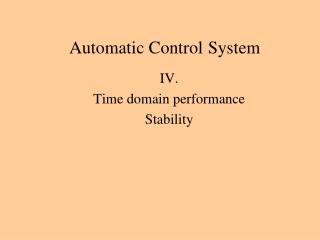DownloadDownload PresentationAutomatic Control System

# Automatic Control System

Download Presentation## Automatic Control System

- - - - - - - - - - - - - - - - - - - - - - - - - - - E N D - - - - - - - - - - - - - - - - - - - - - - - - - - -
##### Presentation Transcript

1. Automatic ControlSystem IV. Time domain performance Stability

2. Ga(s) Transfer functions of closed loop Gw(s) Gr(s) Gp1(s) Gp2(s) Gc(s) Gt(s)

3. y(t) t Steady-state characteristic of the feedback system Y WP2 WP1 Dynamic response of the feedback system R The unit step response of the feedback systems shows the quality parameters in time domain. r(t) t

4. Time domain performance specification (rv-fv) = Steady-state error 90% Tolerance band Second peak value (spv) Settling time Rise time 10% Final value (fv) of response It’s 100% Peak value (pv) Required value of response (rv)

5. Stability of a closed loop system Definition of stability of linear system: Energised the closed-loop system input a short impulse which move the system from it’s steady-state position and wait. When the transient response has died and the system remove the original positionor remain inside a predicted defined small area of the original position the system is stable and linear. Definition of stability of non-linear system: Energised the closed-loop system input a short impulse which move the system from it’s steady-state position and wait. If the system remove and remain inside a predicted defined small area of the original position the system is stable. If the transient response hasn’t died the system is unstable.

6. Stability examination from closed loop transfer function Unit feedback model r(s) y(s)

7. Continuation of stability examination from closed loop The transient solution is given bz the roots of the differential equation’s characteristic equation. The roots are equal the denominator of closed loop transfer function and so it gives transient solution too.

8. Definition of stability in frequency domain The system is stable if the all real part of the rootsof denominator of the transfer function is negative. The pi are named the poles and zj are named the zeros

9. Stability examination from closed loop’s transfer function The root locus diagram Poles and zeros arrangement The root loci depends on the arrangement of poles and zeros and changing a parameter. The default: K runs from zero value to infinite. The loci shows the actual the roots’ positions at actual parameters’ value. Poles arrangement of actual value of K

10. Advantages of stability examination from closed loop’s transfer function Poles and zeros arrangement Minimum damping ratio ofthe second order parts of transfer function Acceptable region for poles Using the results derived for first andsecond-order systems enables a boundeddesign region to be drawn on the s planewithin which the poles produce anacceptable performance. Maximum settling time

11. Stability examination from transfer function of opened-loop Opened-loop transfer function From definition of stability: The frequency at which the open-loop system gain is unity is termedthe gain-crossover frequency. The frequency at which the open-loop system phase-shift is -180° is termed the phase-crossover frequency. The system is stable if plots of the opened-loop transfer functionat the gain-crossover frequency the phase-shift less than -180° andat the phase-crossover frequency the amplitude gain less than unity.

12. Gain margin and phase margin Opened-loop transfer function The gain margin is the reciprocal of open-loop gain at the phase-crossoverfrequency. Expressed in decibel the gain margins is: -20log(open-loop’s gain at the phase-crossover frequency) dB The phase margin is the difference of the phase-shift of the system and -180° at the gain-crossover frequency. The system is stable if plots of the opened-loop transfer function at thegain-crossover frequency has a positive the phase margin and at the phase-crossover frequency has a positive the gain margin.

13. Stability examination from transfer function of opened-loop Independent plots the amplitude gainand phase-shift on lg axis is namedBode diagram. It is the x axis line on the amplitude plotsand the -180 phase-shift line on thephase-shift plots. If the gain and phase margin criterion is true the system is stable. Plots on j (s) planeis named Nyquist diagram. This is a point on the s plane. If the area of the s plane whichis bordered by the positive realaxis and the Nyquist plotsdoesn’t cover this point than the system is stable.

14. Advantages of working with Bode plots Bode form of opened-loop transfer function Bode plots of systems in series simply add.Bode’s important phase-gain relationship is given.A much wider range of system behaviour can be displayed on a single plot.Dynamic compensator design can be based on Bode plots.

15. Using MATLAB Start of stability examination K=1 First using root’s loci. Enabled damping ratio: 0.72, 0.45, 0,23 Secondly using Bode plots. Enabled phase shift: -120, -135, -150 Define the time domain performance specifications.

16. Summary questions • Definition of stability of linear and non-linear systems. • Which methods have used the closed-loop transfer function to examine the stability a control system? • Which methods have used the opened-loop transfer function to examine the stability a control system? • Explain the following terms: phase margin, gain margin. • Explain what is meant by the term “dead time” in control and how it may affect the stability of a control loop.